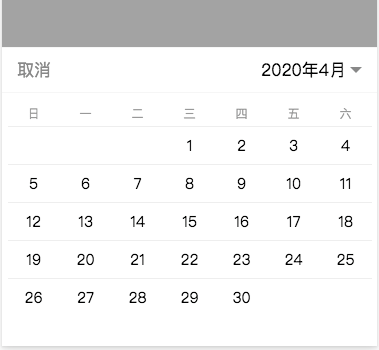# 置顶日历形式的日期选择器精选热门``````if (week > 0) {
for (var i = 0; i < week; i++) {
days.push({
day: 0
})
}
}
``````

``````if (month == 4 || month == 6 || month == 9 || month == 11) {
max = 30
} else if (month == 2) {
if (((year % 4) == 0 && (year % 100) > 0) || (year % 400) == 0) {
max = 29
} else {
max = 28
}
}
``````

5 个评论